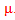Measures of Center

A measure of center is a value at the center or middle of a data set. Graphically, the center can be viewed as the "balance point" of the display. Algebraically, the most common ways to find the center are with the mean, median, or mode. Mean is the average or sum of all data points divided by the number of points. The notation used for mean is the greek letter mu,. Median is the middle value. In order to find the median, put the points in increasing order and find the middle value. If there is an even number of data points, find the average between the two middle points. The notation used for median is a capital letter m, M. Mode is the most often occurring number.